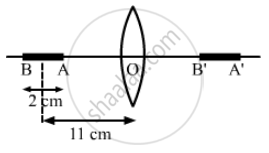Department of Pre-University Education, KarnatakaPUC Karnataka Science Class 12
Advertisement Remove all ads

# A Pin of Length 2.0 Cm Lies Along the Principal Axis of a Converging Lens, the Centre Being at a Distance of 11 Cm from the Lens. - Physics

Sum

A pin of length 2.0 cm lies along the principal axis of a converging lens, the centre being at a distance of 11 cm from the lens. The focal length of the lens is 6 cm. Find the size of the image.

Advertisement Remove all ads

#### Solution

Given,
Length of the pin = 2.0 cm
Focal length (f) of the lens = 6 cm
As per the question, the centre of the pin is 11 cm away from the lens.
i.e., the object distance (u) = 10 cmSince, we have to calculate the image of A and B, Let the image be A' and B'
So, the length of the A'B' = size of the image.
Using lens formula: $\frac{1}{v_A} - \frac{1}{u_A} = \frac{1}{f}$
Where vA and uA are the image and object distances from point A.
$\Rightarrow \frac{1}{v_A} - \frac{1}{- 10} = \frac{1}{6}$
$\frac{1}{v_A} = \frac{1}{6} - \frac{1}{10} = \frac{1}{15}$
$v_A = 15 \text{ cm }$
Similarly for point B,
Lens formula: $\frac{1}{v_B} - \frac{1}{u_B} = \frac{1}{f}$
Where vA and uA are the image and object distances from point B.

$\frac{1}{v_B} - \frac{1}{- 12} = \frac{1}{6}$

$\Rightarrow v_B = 12 \text{ cm }$

Length of image = vA − vB = 15 − 12 = 3 cm.

Concept: Refraction at Spherical Surfaces and by Lenses - Refraction by a Lens
Is there an error in this question or solution?
Advertisement Remove all ads

#### APPEARS IN

HC Verma Class 11, 12 Concepts of Physics 1
Chapter 18 Geometrical Optics
Q 58 | Page 416
Advertisement Remove all ads
Advertisement Remove all ads
Share
Notifications

View all notifications

Forgot password?